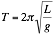## SHM in a Pendulum

SHM in a Pendulum

The motion of a simple pendulum is very close to Simple Harmonic Motion (SHM). SHM results whenever a restoring force is proportional to the displacement, a relationship often known as Hooke’s Law when applied to springs.

F = -kx

Where F is the restoring force, k is the spring constant, and x is the displacement.

Using Newton’s Second Law, the resulting acceleration when there are no other forces, this relationship becomes

ma = F = -kx

where m is mass and a is acceleration.   The result is that the acceleration vector is proportional to the displacement (and in an opposite direction).

When an object is in SHM, is follows the path of a Sine wave.How could you view this sinusoidal motion in a pendulum?

One way would be to use an ultrasonic motion sensor to view the position of the pendulum in real time.

Another way would be to use a wireless accelerometer.  Here is an example of the acceleration of a pendulum taken with a wireless accelerometer, the WDSS made by Vernier.My favorite way would be to use image analysis.  One could use a webcam to gather video footage of the pendulum.  You could analyze the position step-by-step using Vernier LoggerPro or Video Physics.   There are LabView VIs that would allow you to do the image analysis.

Here is a cool HTML version of the Lab View image analysis that was developed at the Tufts CEEO.  Note, you need to use Chrome for your browser.  Check out the pendulum bowling ball from the BUA back stairwell. Using this page, draw a circle on the bowling ball and adjust the threshhold value.  Then hit the Track button!  You can actually export the data in a Google Doc.

In the case of a pendulum, if the amplitude of these cycles are small (q less than 15 degrees) then we can use the Small Angle Approximation for the pendulum and the motion is nearly SHM. A graph of the position of a pendulum as a function of time looks like a sine wave.  You can observe the sinusoidal motion of a pendulum in a Physlet by Andrew Duffy of Boston University.

This model for the period of a pendulum only applies for the small angle approximation.  As the amplitude becomes greater than 10 degrees, the period deviates from this equation.  The motion is no longer sinusoidal as shown in the Physlet.

The reason this approximation works is because for small angles, SIN θ ≈ θ.
This explanation can be defined using the Taylor Series expansion for the SIN of θ where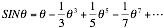For small angles (in units of radians) the powers of θ become increasingly smaller, thus the higher order terms in the Taylor series vanish.

So we can use the small angle approximation in analyzing the pendulum using Newton’s Laws.

# FORCE ANALYSIS

There are two forces on the pendulum, gravity and the tension due to the string.

We can analyze the motion of the pendulum using Newton’s 2nd law in Rotational Coordinates.   In this case the tension is always perpendicular to the motion of the pendulum.  Thus although the tension changes the direction of the pendulum, it does not change the speed of the pendulum.The restoring force on a pendulum is caused by gravity.  Incidentally, because of the pendulum’s own inertia, you will see that the mass of the pendulum cancels out of the equations, and the period of a pendulum is independent of mass.

As gravity points down, we need to take the component of gravity which is parallel to its motion.   Only the component of gravity which is parallel to the direction of motion will do work.   In this case, the force on a pendulum can be given as

F= g SINθ

If we were to examine the torque on the pendulum, we could express this as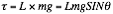where τ is the Torque and L is length of the pendulum.

Using Newton’s second law in rotational coordinates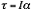where I is the rotational inertia or moment of inertia given by I = mL2, and α is the angular acceleration.  In this case we can say that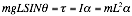and a factor of m and L both cancel out, reducing to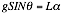Using the small angle approximation SINθ ≈ θ, this equation is approximately

gθ=Lα

or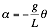Here we have the conditions for Simple Harmonic Motion where the angular acceleration is proportional to the angular displacement.  The solution for this equation is that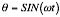where the angular frequency ω is proportional to the linear frequency f as given by

ω=2πf

The frequency  f is measured in cycles per/second and the angular frequency is usually measured in radians per second.

Using calculus and taking the second derivative of our expression for angular position we find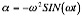Plugging this into

gθ=Lα

we find that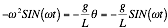As the sine term cancels, giving us an expression of the angular frequency in terms of gravity and length.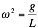Using the definition of the angular frequency and the reciprocal relationship between period of time and frequency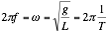So the period of the pendulum is proportional to the square root of the length of the pendulum, and inversely proportional to the square root of the acceleration due to gravity.